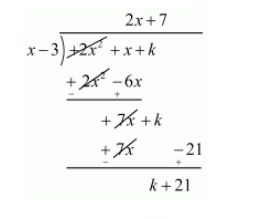# For what value of k, is 3 a zero of the polynomial 2x2 + x + k?`
Question:

For what value of $k$, is 3 a zero of the polynomial $2 x^{2}+x+k ?$

Solution:

We know that if $x=\alpha$ is zero polynomial, and then $x-\alpha$ is a factor of $f(x)$

Since 3 is zero of $f(x)$

Therefore $x-3$ is a factor of $f(x)$

Now, we divide $f(x)=2 x^{2}+x+k$ by $g(x)=x-3$ to find the value of $k$Now, remainder $=0$

$k+21=0$

$k=-21$

Hence, the value of $k$ is $-21$